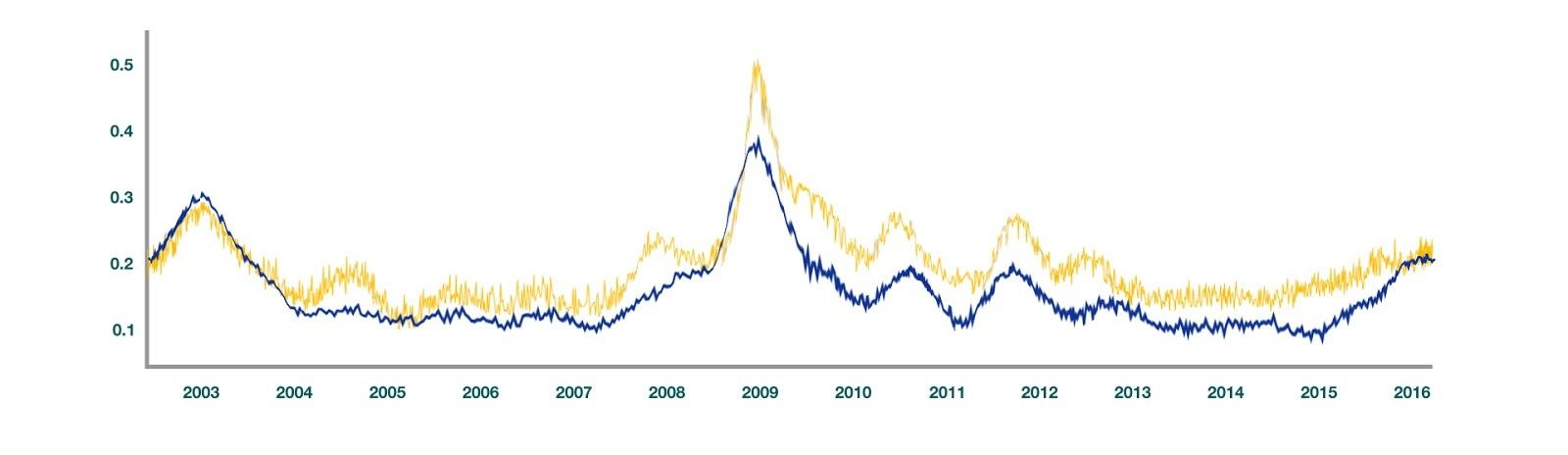# Implied Volatility: What, Why & How!

“When a long-term trend loses its momentum, short-term volatility tends to rise.” - George Soros

Well, the quote sounds interesting and captivating, but how do you know what is the market’s expectation of volatility? Moreover, is there a way to calculate future volatility which would help us in taking long or short positions in the options trading space? Sounds intriguing, right!!

Well, we have got all your questions related to “Implied Volatility in options trading” covered in this article. The term used to characterize expected market volatility from the date when the option is bought till its expiry by market participants is known as “Implied Volatility (IV)”. But before we jump into the peculiar and distinct characteristics of implied volatility, let’s take a look into the sub-topics being covered for this article.

## Understanding Implied Volatility

Before we dive into the basics of implied volatility, you should be aware of the options trading basics.

Index Option trading model based on Index’s momentum

EPATian's real trading project with data and Python code

### Volatility:

Volatility is one of the most important pillars in financial markets. In simple words, volatility refers to the upward and downward price movements of a financial asset. The movements are due to several factors including demand and supply, sentiment, corporate actions, greed, and fear, etc.

Now that we know what volatility is, let us now understand what implied volatility really means!!

### What is Implied Volatility?

Implied Volatility (IV) is the measure of expected future volatility in the options market. Essentially, implied volatility was and is still considered to be an integral component of the Black-Scholes-Merton model (a popular option pricing model), where it represents future volatility associated with the underlying asset.

But, did you know that it is not the only type of volatility measure available in the market? Historical and realized volatility are other different types of volatility measures in the market.

#### Historical volatility

Historical volatility indicates the deviation or change in prices of the underlying asset over a given period of time in past. Usually, historical volatility is calculated over a period of one-year i.e. 252 trading days. It is used by traders to compare the current volatility level of an underlying asset with its historical volatility. Whenever there is a gap between the current and historical volatility, traders take positions based on the opportunity. However, the issue with historical volatility is that it is a backwards-looking indicator which means it is based on the past returns and is not the most reliable form of volatility.

#### Realized Volatility

Realized volatility, on the other hand, is the actual volatility that will take place in the future. For the volatility, that has taken place in the past, it is known as historical volatility and for the volatility that will take place in the future, it is known as realized volatility.

So, why do we use implied volatility in the options market?

The value of implied volatility has been factored in after considering market expectations. Market expectations may be major market events, court rulings, top management shuffle, etc. In essence, implied volatility is a better way of estimating future volatility in comparison to historical volatility, which is based only on past returns.

## Math behind IV

We will now move forward and understand the mathematics behind Implied Volatility and how it is calculated for options.

Calculating IV is not an easy task as it might appear to be. To calculate the Implied Volatility of a call or put option, we first need to understand the mathematics behind the Black Scholes Merton(BSM) Model. As for the purpose of this article, we will not dig down much into the concept of the BSM Model but we will definitely have an overview of what is the BSM model so that the calculation of Implied Volatility looks similar and easy to understand.

### Black Scholes Merton Model:

The Black-Scholes-Merton model is the most popular option pricing model used by traders when it comes to European options. It has two separate formulas for calculating the call option and the put option.

Index Option trading model based on Index’s momentum

EPATian's real trading project with data and Python code

The Parameters for calculating the call option are :

• St – Spot Price of the underlying asset (Current Price)
• K – Strike Price of the underlying asset
• r – Risk-free rate(continuously compounded)
• σ – Volatility of returns of the underlying asset
• T-t – Time to maturity (in years)
• N – Cumulative distribution function of Normal Distribution

Pricing the call option :Pricing the put option :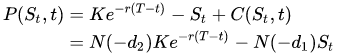Looks a bit complicated right? Don’t worry, once you input the values of the parameters it is just like any other simple equation.

For example: If the parameters are as follows :

Spot Price (St): 300 Strike Price (K): 250 Risk-free rate (r) = 5% Time to maturity (T-t) = 0.5 years (6 months) Call Price = 57.38

How do we find the implied volatility for the call option with the parameters as mentioned above? We will simply use the method of reiteration or trial and error.

Using the IV of 15% fetches us a call option price of 56.45 and using 25% gives us a call price of 59.

It is clear from the above trial and error method that the IV is a value between the range of 15 - 25%. What about 20?. It gives us a call price of 57.38!! The same technique can be used for put options accordingly. Once you get hold of this technique, it's easy as pie!

## Calculating IV using python

Alright, now that we know the concept of implied volatility, why not create a calculator for calculating IV of an option? After all, the knowledge earned should be applied practically!!

We will create an implied volatility calculator using python for easy calculation of IV for an option.

Index Option trading model based on Index’s momentum

EPATian's real trading project with data and Python code

The Python Code :

## Let us first import all the required libraries for IV Calculation.
# Data manipulation
import numpy as np
import pandas as pd
import datetime
import mibian

# We will now use the mibian library to calculate the implied volatility.
##The syntax for the variable values is in the format as mentioned below :
# BS([UnderlyingPrice, StrikePrice, InterestRate, Daystoexpiration],callPrice=x)

# Python code :
c = mibian.BS([145.65, 145, 5, 30], callPrice=3.89)

# Input Code :
c.impliedVolatility

Output for the input code :

18.24951171875

This means that the implied volatility for the call option is 18.249% (approx)

Wasn’t that simple?! Python calculates a complex mathematical model such as Black-Scholes-Merton formula very quickly and easily. This same mechanism can be used to calculate put option implied volatility.

## Factors affecting Implied Volatility in the market

Let’s take a look at certain factors that influence implied volatility in options trading:

Supply and Demand - With the increase in the demand for an underlying asset, the implied volatility increases too and so does the option price! Of course, this phenomenon is exactly the opposite when the demand is low. High IVs tend to move towards the mean IV value with the fall in demand and the supply starts stabilizing concurrently. This all takes place once the market expectation starts falling and leads to a reduction in the option price.

Time to Expiration - Time to expiration, better known as theta, which measures the amount of time left for the option to expire, affects the IV of an option directly. For example, if the time to expiry is little, the IV usually would be on the lower side. However, if the time for the expiration of an option is relatively longer than usual, IV would be high. Logically, it makes sense too! How? Since the time to expiration is high, there is a lot more chance that the underlying asset’s price might move towards the strike price and that is too risky for the option seller. To compensate for the risk taken by the seller, the option price is relatively higher than usual and so is the IV.

Market condition - Most underlying assets are directly impacted by the market sentiment or events that are to take place in the future for a listed organisation. Earnings announcement, court ruling, top management shuffle, etc are some of the market events that lead to high IV with an option as the market is unsure of the direction that the underlying asset might move.

Index Option trading model based on Index’s momentum

EPATian's real trading project with data and Python code

## Uses of IV

Implied Volatility is certainly used frequently in the options market by traders for varied reasons. Listed below are the various uses of IV :

To forecast volatility - Implied Volatility is used by traders to understand the range of expected volatility for an underlying asset. For example, let us consider a call option with an underlying asset currently trading at $100, the strike price at$103 and the premium at \$5. If the Implied volatility is 20% for such a call option, the expected range for the underlying asset is 20% above the current trade price and 20% below the current trade price. This tells us that the lower bound would be at 100 - 20% of 100 = 100 - 20 = 80. The upper bound at 100 + 20% of 100 = 100 + 20 = 120. The range of the implied volatility in such a case would be from 80 - 120.

To hedge cash position - A trader frequently needs to hedge a position to reduce the risk associated with the initial or primary position. If the current IV of an option is comparatively lower than the annualized IV or the IV for the entire year, a trader can buy options at a low premium and wait until the IV increases. With the increase in IV, the value of the option premium rises too and thereby the total value of the option contract jumps up!

To write options - Contrary to hedging, option writers (option sellers) sell options when the IV is high and thereby pocket high premiums for the risk they are undertaking. The catch here is that for the insurance (option) they are selling, time to expiration keeps decreasing. After a considerable time period has elapsed, the trade moves into the favour of the option seller.

Event-based trading - Whenever there is news relating to earnings or court ruling pending for a listed organization, the IV is usually high. This happens when the future is likely to be uncertain. In such a scenario, informed or experienced traders do create option strategies revolving around implied volatility. For example, traders use calendar spread strategy, bull or bear spread strategy to benefit from high IV.

Usage in Black -Scholes-Merton (BSM) Model: Implied Volatility is a key parameter when it comes to BSM Model. As the implied volatility or the market expectation about the volatility increases, the option price increases. This creates a direct relationship between implied volatility and the option price. IV, therefore, forms an intrinsic property of the Black Scholes Merton Model.

## Interpreting IV

There is more than one way to visualize and interpret implied volatility and we will look at each one of them specifically.

Index Option trading model based on Index’s momentum

EPATian's real trading project with data and Python code

Data Table - Well, the most basic way to visualize IV numbers would be through a data table format. Now, in the options market, it is known as an option chain. Below is an Option Chain for the US Stock: Apple (ticker: AAPL)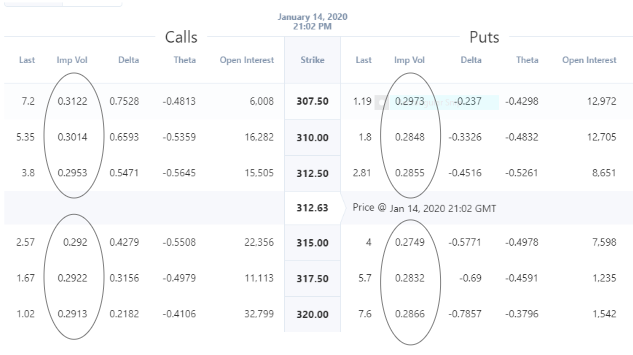Source: Investing.com ( Note: Options data is updated each day, so you will be able to see the current’s day option prices on the given link)

From the above image, it is very clear that the Implied Volatility for the same strike price is different for call and put options. Also, for different strike prices, the Implied Volatility fluctuates with the shift in market expectations. Note: Implied Volatility is not a direction based parameter and therefore it only indicates the range of prices an underlying asset might move in the future. This change in implied volatility in both the put and call option at different strike prices is characterized by "Volatility Smile" and Volatility Skew. Volatility Smile takes place when the implied volatility(IV) is the highest at OTM and ITM call or put options with the lowest at, ATM option. In the case of Volatility Skew, different strike prices have different implied volatility for the same underlying asset.

Both interpretations are used in the options market for better visualization purposes. Below, we have mentioned the Volatility Skew example from the call option strike prices and implied volatility relatively.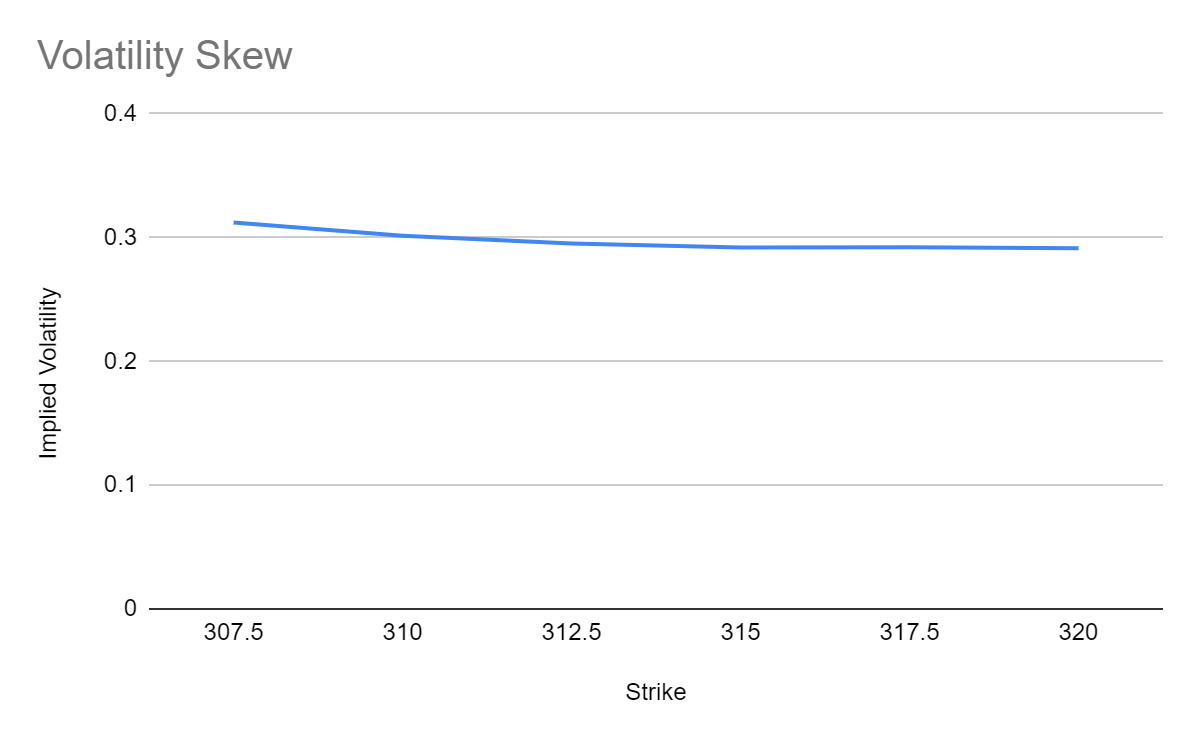Chart - Alright, now that we have understood and interpreted implied volatility from an options chain data table, we will visualize implied volatility through a chart and interpret IV levels from the same.

Index Option trading model based on Index’s momentum

EPATian's real trading project with data and Python code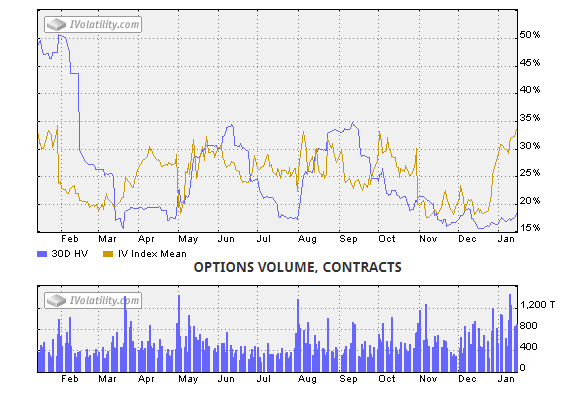Source: IVolatility.com

In the chart, we have the implied as well as 30-Day historical volatility data for the past one year.

Market participants, use historical implied volatility levels to gauge an understanding of where the IV, say, for example, was at 3 months ago and at what level it is today for trading based on the opportunity.

Traders also use past trends of both historical and implied volatility to understand if the HV and IV together are higher or lower than previous periods. If you start trading options today, this is your go-to tool for gauging implied volatility levels. As stated earlier, there are a number of factors why the implied volatility level is high or low at a certain point in time.

Implied Volatility (IV) Rank - IV Rank is another popular way of calculating the implied volatility over the last one year or 52 weeks. It is calculated for figuring out how high or low the current IV level is when compared with the annualized levels. There is a particular formula to calculate IV Rank which is mentioned below:

(Current IV - 52 weeks low IV / 52 week high IV - 52 weeks low IV) * 100

For Example: Let’s consider the example of Apple (ticker: AAPL) which was mentioned in the chart section of IV. The current IV is at 32.5%, 52 week low IV is 18%. 52 week high IV is 34%. So let’s do the math :

32.5% - 18% / 34% - 18% = 14.5% / 16% = 90.625%.

Interpreting the IV Rank is easy too. Intuitively IV rank refers to the difference between the Current IV and 52 week low IV i. In this case, it is 90.625%. This means that the IV is currently higher than usual and a trader would be interested in selling the options due to high IV. High IV means high option price and thus would benefit the option sellers heavily. Option buyers who buy options with high IV face losses due to the decrease in IV at a later point in time.

Implied Volatility (IV) Percentile - IV Percentile is another interesting way to look at IV or to interpret it. IV Percentile simply refers to the number of days the current IV is under the current IV percentage value as compared to the total number of trading days .i.e. 252 trading days

IV Percentile = Number of trading days under current IV / Number of trading days in a year.

For example: If the number of days under the current IV (30%) is 100. The number of trading days is 252. IV Percentile = 100/252 = 39.68 percentile (approx).

Below is a data table of Indian Stocks dated for the 26th of November, 2019 with their IV Rank and IV Percentile for visualizing IVR and IVP!

Index Option trading model based on Index’s momentum

EPATian's real trading project with data and Python code

 Symbol IV Rank IV Percentile Implied Volatility TATACOMM 86.99 98.47 48.2 SUZLON 93.17 98.54 184.13 NATIONALUM 73.74 96.65 61.18 CGPOWER 81.12 96.4 106.29 BOSCHLTD 78.16 87.94 36.65 RAYMOND 71.63 95.43 47.33 IDEA 73.04 96.27 167.51 NBCC 72.05 98.74 90.43 IRB 71.43 93.53 87.38 TV18BRDCST 97.1 97.84 80.15 JETAIRWAYS 100 100 483.73

Let’s us deduce the concept of IVP with relation to Implied Volatility with an example of two equity stocks i.e. Tata Communications Limited (TATACOMM) and Suzlon Energy Ltd(SUZLON). TATACOMM has an Implied Volatility(IV) of 48.2 % whereas SUZLON has an Implied Volatility of 184.13%. Given that there is a huge gap between the IVs of both the equity stock options, to the logical mind, it looks like the IVP should have a huge difference too. However, in reality, the IVP of TATACOMM is 98.47 and for SUZLON, it is 98.54, which makes a difference of only 0.07 percentile!

This means that even if the IV of TATACOMM was at 48.2%, it was still trading at one of its highest levels similar to SUZLON. Therefore, before trading options using IV, one should be aware as to what has been the historical IV values for an option and where it stands currently. This is exactly where the application of Implied Volatility Percentile becomes crucial, where it helps us in identifying current IV values in comparison to where the IV has been over the past one year ( 252 trading days).

Historical IV vs Realized Volatility - Historically, IV has a trend of mostly being more than the realized volatility. Market expectations keep fluctuating which means that they are always either more or less than the realized volatility value of the underlying asset. In the below example, we show the Dow Jones Index’s comparison between Implied Volatility and realized volatility (volatility that actually took place) to visualize the same concept. The Blue line represents realized volatility and the yellow line represents implied volatility. Implied Volatility is mostly above the realized volatility due to fluctuation in market expectations.